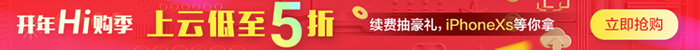1. 云栖社区>
2. Java技术进阶>
3. 博客>
4. 正文## 如何实现逻辑时钟

### Lamport 逻辑时钟

Clock Condition.对于任意事件$a$, $b$：如果$a \to b$（$\to$表示a先于b发生），那么$C(a)< C(b)$, 反之不然, 因为有可能是并发事件
C1.如果$a$和$b$都是进程$P_i$里的事件，并且$a$在$b$之前，那么$C_i(a) < C_i(b)$
C2.如果$a$是进程$P_i$里关于某消息的发送事件，$b$是另一进程$P_j$里关于该消息的接收事件，那么$C_i(a) < C_j(b)$

Lamport 逻辑时钟原理如下：1. 每个事件对应一个Lamport时间戳，初始值为0
2. 如果事件在节点内发生，本地进程中的时间戳加1
3. 如果事件属于发送事件，本地进程中的时间戳加1并在消息中带上该时间戳
4. 如果事件属于接收事件，本地进程中的时间戳 = Max(本地时间戳，消息中的时间戳) + 1

## 如何使用逻辑时钟解决分布式锁问题

1. 已经获得资源授权的进程，必须在资源分配给其他进程之前释放掉它；
2. 资源请求必须按照请求发生的顺序进行授权；
3. 在获得资源授权的所有进程最终释放资源后，所有的资源请求必须都已经被授权了。

1. 为请求该项资源，进程$P_i$发送一个$(T_m:P_i)$资源请求（请求锁）消息给其他所有进程，并将该消息放入自己的请求队列，在这里$T_m$代表了消息的时间戳
2. 当进程$P_j$收到$(T_m:P_i)$资源请求消息后，将它放到自己的请求队列中，并发送一个带时间戳的确认消息给$P_i$。(注：如果$P_j$已经发送了一个时间戳大于$T_m$的消息，那就可以不发送)
3. 释放该项资源（释放锁）时，进程$P_i$从自己的消息队列中删除所有的$(T_m:P_i)$资源请求，同时给其他所有进程发送一个带有时间戳的$P_i$资源释放消息
4. 当进程$P_j$收到$P_i$资源释放消息后，它就从自己的消息队列中删除所有的$(T_m:P_i)$资源请求
5. 当同时满足如下两个条件时，就将资源分配（锁占用）给进程$P_i$：

• 按照全序关系排序后，$(T_m:P_i)$资源请求排在它的请求队列的最前面
• $i$已经从所有其他进程都收到了时间戳>$T_m$的消息、## 参考资料

【云栖快讯】一站式开发者服务，海量学习资源免费学  详情请点击

+ 关注